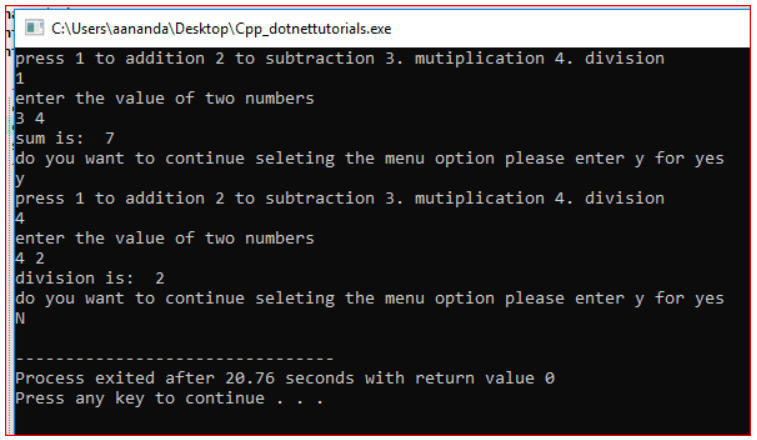# Do While Loop in C++

## Do While Loop in C++ with Example:

In this article, I am going to discuss Do While Loop in C++ Language with definitions, syntax, flow charts, and examples. Please read our previous articles, where we discussed While Loop in C++ Language with Examples. At the end of this article, you will understand what is the do-while loop and when and how to use a do-while loop in the C++ program with examples.

##### What is Do while loop in C++?

The do-while loop is a post-tested loop. Using the do-while loop, we can repeat the execution of several parts of the statements. The do-while loop is mainly used in the case where we need to execute the loop at least once. The do-while loop is mostly used in menu-driven programs where the termination condition depends upon the end-user.

##### Do-while Loop in C++:

Following is the do While loop syntax in C++:
do {
// process
} while (<condition>);

This is the syntax of the do-while loop. In the curly braces, we will write the statements which we want to process repeatedly and after closing the curly bracket, we will write while and give the condition of termination in the parenthesis. This condition must end with a semicolon. We don’t use the semicolon in the while loop but it is a must in do-while.

Note: do-while first process and then check the condition.

##### Flowchart of do-while loop in C++: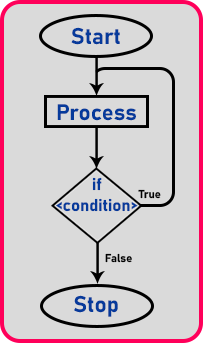In the do-while loop, first, it will perform processing without checking the condition. After processing, it will check for condition, if the condition is true then control goes to the process part as shown in the flowchart and if the condition is false then it will stop here, and control come out of the loop.

##### While and do-while are almost the same. So, what is the difference? Which one do we use?

We can use any one of the loops. Only the difference is, in the while loop if the already condition will be false then it will not perform any processing, it will not execute the statement under curly braces. But in the do-while, if the already condition will be false then it will first perform processing and then check the condition. It means, at false condition do-while will be run for at least one time.

Note: When you want to execute the loop body at least once irrespective of the condition, then you need to use the do-while loop.

##### Program to print numbers from 1 to n using a do-while loop:

Let us first look at the flowchart: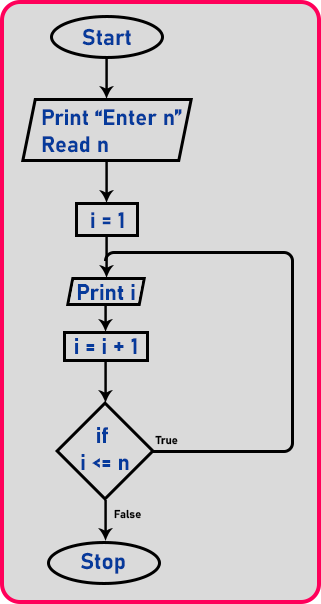1. Step 1: First, we will take the input as far as we want to print the number.
2. Step 2: To print the numbers, we need a counter, so here we have ‘i’ as a counter. So we have initialized ‘i’ to 1. So ‘i’ starts from one onwards.
3. Step 3: Next it will print ‘i’ and then increment ‘i’ as ‘i = i + 1’. After this, it will check for the condition. If (i <= n) is true then it will go back to the previous statements and execute them again. If the condition fails then it will stop the loop.

Note: Do While Loop is Popularly known as a post-tested loop. The behavior is the same, as while loop with only difference loop is executed first then it will check the condition. This behavior of checking the condition last ensures the loop gets executed at least once. This is also an example of a conditional loop and the condition is tested after executing the loop body.

##### Program to Print numbers from 1 to n using do-while loop in C++ language:
```#include <iostream>
using namespace std;
int main()
{
int n, i = 1;
cout << "Enter number:" << endl;
cin >> n;
cout << endl;
do
{
cout << i << endl;
i++;
}
while (i <= n);
return 0;
}
```
###### Output: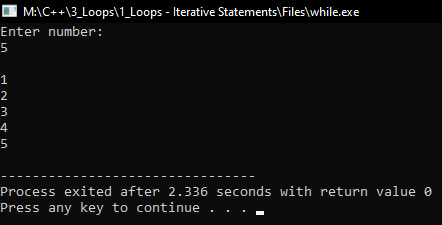##### Program to print one statement for n time using a do-while loop:
```#include <iostream>
using namespace std;
int main()
{
int n, i = 1;
cout << "Enter number:" << endl;
cin >> n;
cout << endl;
do
{
cout << "Hello C++" << endl;
i++;
}
while (i <= n);
return 0;
}
```
###### Output: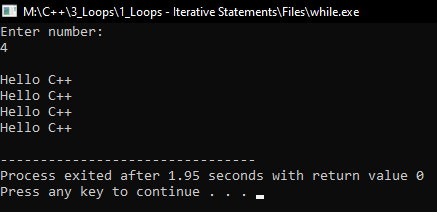##### Nested do-while Loop in C++:

Using a do-while loop within do-while loops is said to be a nested do-while loop. The syntax to use the nested do-while loop in the C++ language is given below.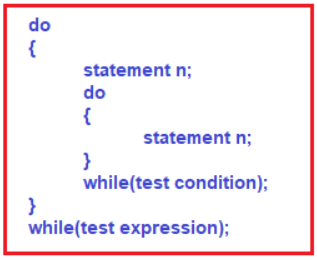##### Program to understand Nested Do While Loop in C++:
```#include <iostream>
using namespace std;
int main()
{
do
{
cout << "I'm from outer do-while loop ";
do
{
cout << "\nI'm from inner do-while loop ";
}
while (1 > 10);
}
while (2 > 10);
return 0;
}
```
###### Output: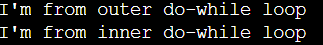##### Use case scenario of the do-while loop in C++:

The do-while loop is used in menu-driven programs. To better explain this, consider the program below.

```#include <iostream>
using namespace std;
int main()
{
char choice;
int a, b;
do
{
cout <<"press 1 to addition 2 to subtraction 3. mutiplication 4. division" << endl;
{
case 1:
cout << "enter the value of two numbers" << endl;
cin >> a >> b;
cout << "sum is:" << "  " << a + b << endl;
break;
case 2:
cout << "enter the value of two numbers" << endl;
cin >> a >> b;
cout << "difference is:" << "  " << a - b << endl;
break;
case 3:
cout << "enter the value of two numbers" << endl;
cin >> a >> b;
cout << "multipy is:" << "  " << a * b << endl;
break;
case 4:
cout << "enter the value of two numbers" << endl;
cin >> a >> b;
cout << "division is:" << "  " << a / b << endl;
break;
default:
cout << "invalid choice" << endl;
}
cout <<"do you want to continue seleting the menu option please enter y for yes"<< endl;
cin >> choice;
}
while (choice == 'y');
return 0;
}
```
###### Output: